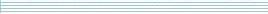| home      | people     | research     | publications    | seminars    | events     | contactCommunication Networks   | Systems Biology   | Hybrid Systems    | Machine Learning   | Dynamics & Interaction### Schools Mathematics Grand Challenge

Week six's Puzzles

Problem 11:

The eleventh problem was:

There is exactly one three digit positive whole number (so a number between 100 and 999) with the following properties:

If you subtract 11 from it, the answer is divisible by 11;
If you subtract 10 from it, the answer is divisible by 10;
If you subtract 9 from it, the answer is divisible by 9.

What is the number?

The solution was:

If a number minus 11 is divisible by 11 then so is the number itself. The same is true if the number minus 10 is divisible by 10 or the number minus 9 is divisible by 9. So we are looking for a number divisible by 9, 10 and 11. The least common multiple of 9, 10 and 11 is

9 x 10 x 11 = 990

and this is the smallest number with this property. The next smallest such number is 2 x 990 = 1980 which is larger than 1000 so the answer is 990.

Problem 12:

The twelvth problem was:

There are 70 students in a year, 36 girls and 34 boys. At the end of the school year, the students have a choice of two places to go on their school trip: either Galway or Cork. All of the students go on the trip with 25 students going to Galway, and the other 45 going to Cork. How many more girls went to Cork than boys went to Galway?

How many more girls went to Cork than boys went to Galway?

(We want the difference between the number of girls who went to Cork and the number of boys who went to Galway)

The solution was:

Let N be the number of girls who went to Cork and K be the number of boys who went to Galway. We want to know the value of N - K.

Now if K boys went to Galway, and there are 34 boys in the year then the other 34 - K went to Cork. This means that the total number of students (girls and boys) who went to Cork is

N + 34 - K.

But we know that 45 went to Cork so

N + 34 - K = 45

and N - K = 45 - 34 = 11. So the answer is 11.